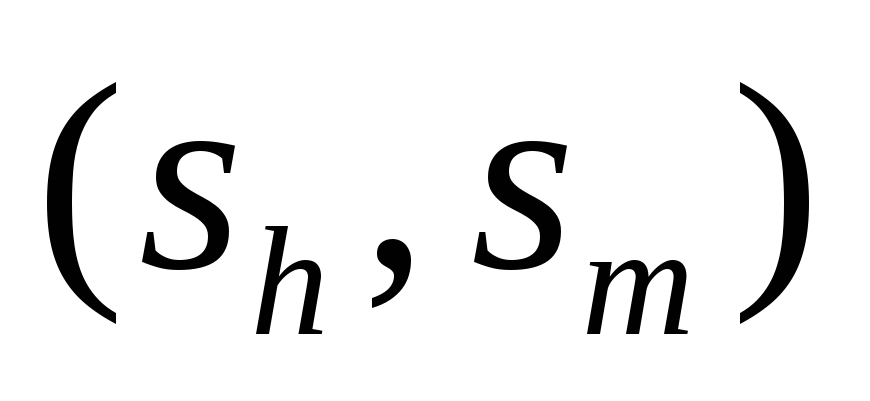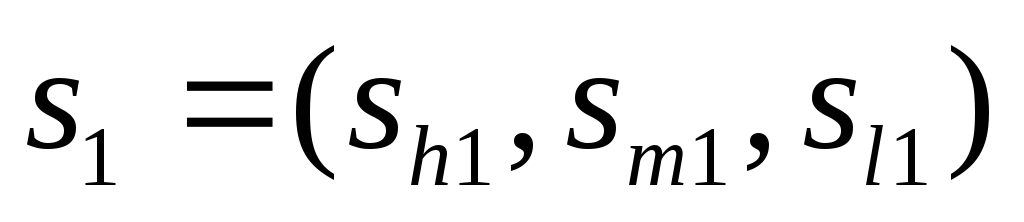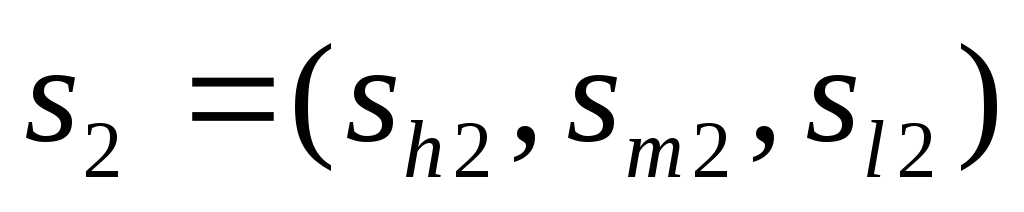# Essays on applied economics

 Lemma 3.3. Starting from the same point, the path (whenis small) under CN is above the path under NCN. We may wonder if this is always true, or the two paths may cross at a later point. Lemma 3.4. After starting from the same point, the path under CN will always be above the path under NCN. Proof: Suppose that at a later point, the two paths reach a same composition. Starting from this point, the path under CN will again be above the path under NCN. So the two paths can never cross. Therefore the path of convergence under CN in theplane is always above the one under NCN. See an example in figure 3 of the simulation. Lemma 3.5. For two population compositionsand, ifand, then the increase rate ofat pointunder CN is less than the increase rate ofat pointunder NCN. Download 2.41 Mb.Share with your friends: